## Practice Questions – Misc Aptitude – 2

1. 40 % of 15 % of ¾ th of a number is 153. What is the number?

(a) 3400

(b) 3650

(c) 3600

(d) 3200

(e) None of these

2. What is 786 times 964?

(a) 759276

(b) 749844

(c) 75416

(d) 757704

(e) None of these

3. If 46is subtracted from the square of a number, the answer so obtained is 485. What is the number?

(a) 49

(b) 51

(c) 56

(d) 53

(e) None of these

4. In an examination it is required to get 40 % of the aggregate marks to pass. A student gets 265 marks and is declared fail by 55 marks. What is the maximum aggregate marks a students can get?

(a) 800

(b) 750

(c) 650

(d) Cannot be determined

(e) None of these

5. The sum of four consecutive even numbers A, B, C and D is 180. What is the sum of the set of next four consecutive even numbers?

(a) 214

(b) 212

(c) 196

(d) 204

(e) None of these

6. In the numerator of a fraction is increased by 200 % and the denominator of the fractions is increased by 150 %, the resultant fractions are 9/35. What is the original fractions?

(a) 3/10

(b) 2/15

(c) 3/16

(d) 2/7

(e) None of these

7. In an annual examination Hardik got 500 marks out of 725. What is his approximate per cent in the examination?

(a) 88

(b) 79

(c) 54

(d) 62

(e) 70

8. The average age of a women and her daughter is 21 yr. The ratio of their ages is 5 : 1 respectively. What will be the ratio of their ages after 5 yr?

(a) 10 : 3

(b) 5 : 2

(c) 4 : 1

(d) 3 : 1

(e) None of these

9. Find the average of the following set of scores.

354, 231, 777, 560, 892, 443, 546

(a) 545

(b) 540

(c) 542

(d) 548

(e) None of these

10. The cost of 4 bowls and 6 bottles is Rs. 142.5. What will be the cost of 8 bowls and 12 bottles?

(a) Rs. 445

(b) Rs. 285

(c) Rs. 345

(d) Cannot be determined

(e) None of these

1. (a) 2. (d) 3. (b) 4. (a) 5. (b) 6. (e) 7. (e) 8. (a) 9. (c) 10. (b)

Explanation

1. Let the number be x.

According to the question,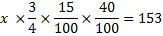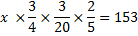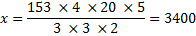2. Required number = 786 × 964 = 757704

3. Let the number be x.

According to the question,

X2 – (46)2 = 485

X2 – 2601= 485

X2 = 2116 + 485 = 2601

X = 51

4. Let the maximum marks be x.

According to the questions,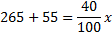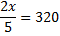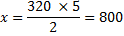5. Let the smallest even number be x.

According to the question,

X + x +2 + x +4 + x + 6 = 180

4x + 12 = 180

4x = 168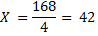X + 6 = 42 + 6 = 48

Required sum = 50 + 52 + 54 + 56 = 212

6. Let the original fraction be x/y.

According to the question,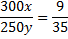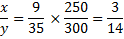7. Required percentage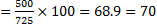8. Let the percentage ages of the women and her daughter be 5x and x yr respectively.

According to the question,

5x + x = 21 × 2

6x = 42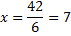Woman’s age = 5 × 7 = 35 yr

Required ratio = (35 + 5): (7 +5) = 40 : 12 = 10 : 3

9. The average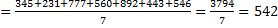10. Let the cost prices of 1 bowl and 1 bottle are Rs. x and Rs. y respectively.

According to the question,

4x + 6y = 142.5

2 (4x + 6y) = 2 × 142.5

8x + 12 y = Rs. 285